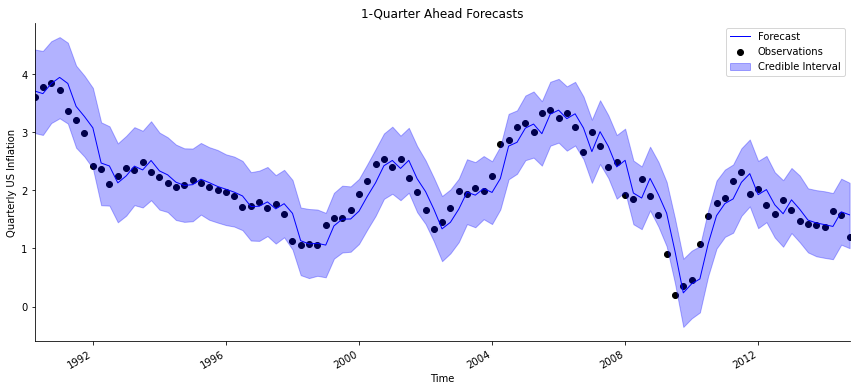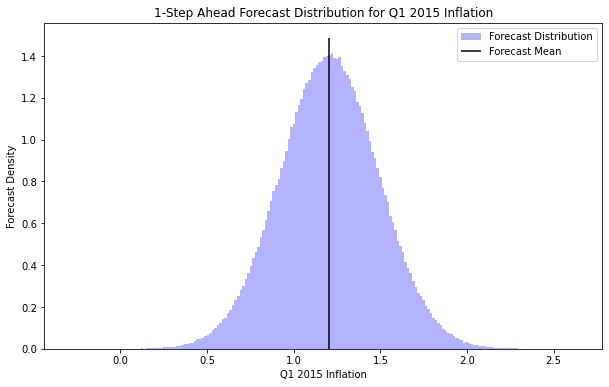The analysis functions help a modeler quickly run a full time series analysis.

An analysis consists of:

1. Initializing a DGLM, using define_dglm.
2. Updating the model coefficients at each time step, using dglm.update.
3. Forecasting at each time step between forecast_start and forecast_end, using dglm.forecast_marginal or dglm.forecast_path.
4. Returning the desired output, specified in the argument ret. The default is to return the model and forecast samples.

The analysis starts by defining a new DGLM with define_dglm. The default number of observations to use is set at prior_length=20. Any arguments that are used to define a model in define_dglm can be passed into analysis as keyword arguments. Alternatively, you may define the model beforehand, and pass the pre-initialized DGLM into analysis as the argument model_prior.

Once the model has been initialized, the analysis loop begins. If $\text{forecast_start} \leq t \leq \text{forecast_end}$, then the model will forecast ahead. The forecast horizon k must be specified. The default is to simulate nsamps=500 times from the forecast distribution using forecast_marginal, from $1$ to k steps into the future. To simulate from the joint forecast distribution over the next k steps, set the flag forecast_path=True. Note that all forecasts are out-of-sample, i.e. they are made before the model has seen the observation. This is to ensure than the forecast accuracy is a more fair representation of future model performance.

After the forecast has been made, the model sees the observation $y_t$, and updates the state vector accordingly.

The analysis ends after seeing the last observation in Y. The output is a list specified by the argument ret, which may contain:

• mod: The final model
• forecast: The forecast samples, stored in a 3-dimensional array with axes nsamps $\times$ forecast length $\times$ k
• model_coef: A time series of the state vector mean vector and variance matrix

Please note that analysis is used on a historic dataset that already exists. This means that a typical sequence of events is to run an analysis on the data you current have, and return the model and forecast samples. The forecast samples are used to evaluate the past forecast performance. Then you can use dglm.forecast_marginal and dglm.forecast_path to forecast into the future.

## Analysis for a DGLM

#### analysis[source]

analysis(Y, X=None, k=1, forecast_start=0, forecast_end=0, nsamps=500, family='normal', n=None, model_prior=None, prior_length=20, ntrend=1, dates=None, holidays=[], seasPeriods=[], seasHarmComponents=[], latent_factor=None, new_latent_factors=None, ret=['model', 'forecast'], mean_only=False, forecast_path=False, **kwargs)

This is a helpful function to run a standard analysis. The function will:

1. Automatically initialize a DGLM
2. Run sequential updating
3. Forecast at each specified time step

This function is core to the PyBATS package, because it allows a modeler to easily run a full time series analysis in one step. Below is a quick example of analysis of quarterly inflation in the US using a normal DLM. We'll start by loading in the data:

from pybats.shared import load_us_inflation
from pybats.analysis import analysis
import pandas as pd
from pybats.plot import plot_data_forecast
from pybats.point_forecast import median
import matplotlib.pyplot as plt
from pybats.loss_functions import MAPE


Date Inflation
0 1977-Q3 6.151037
1 1977-Q4 6.569646
2 1978-Q1 6.445443
147 2014-Q2 1.636442
148 2014-Q3 1.571666
149 2014-Q4 1.189142

And then running an analysis. We're going to use the previous (lag-1) value of inflation as a predictor.

forecast_start = '1990-Q1'
forecast_end = '2014-Q3'
X = data.Inflation.values[:-1]

mod, samples = analysis(Y = data.Inflation.values[1:], X=X, family="normal",
k = 1, prior_length = 12,
forecast_start = forecast_start, forecast_end = forecast_end,
dates=data.Date,
ntrend = 2, deltrend=.99,
seasPeriods=, seasHarmComponents=[[1,2]], delseas=.99,
nsamps = 5000)

beginning forecasting


A couple of things to note here:

• forecast_start and forecast_end were specified as elements in the dates vector. You can also specify forecast_start and forecast_end by row numbers in Y, and avoid providing the dates argument.

• ntrend=2 creates a model with an intercept and a local slope term, and deltrend=.98 discounts the impact of older observations on the trend component by $2\%$ at each time step.

• The seasonal component was set as seasPeriods=, because we think the seasonal effect has a cycle of length $4$ in this quarterly inflation data.

Let's examine the output. Here is the mean and standard deviation of the state vector (aka the coefficients) after the model has seen the last observation in Y:

mod.get_coef()

Mean Standard Deviation
Intercept 0.12 0.08
Local Slope -0.00 0.00
Regn 1 0.91 0.03
Seas 1 0.00 0.03
Seas 2 0.01 0.03
Seas 3 0.00 0.02
Seas 4 -0.00 0.96

It's clear that the lag-1 regression term is dominant, with a mean of $0.92$. The only other large coefficient is the intercept, with a mean of $0.10$.

The seasonal coefficients turned out to be very small. Most likely this is because the publicly available dataset for US inflation is pre-adjusted for seasonality.

The forecast samples are stored in a 3-dimensional array, with axes nsamps $\times$ forecast length $\times$ k:

• nsamps is the number of samples drawn from the forecast distribution
• forecast length is the number of time steps between forecast_start and forecast_end
• k is the forecast horizon, or the number of steps that were forecast ahead

We can plot the forecasts using plot_data_forecast. We'll plot the 1-quarter ahead forecasts, using the median as our point estimate.

forecast = median(samples)

# Plot the 1-quarter ahead forecast
h = 1
start = data[data.Date == forecast_start].index + h
end = data[data.Date == forecast_end].index + h + 1

fig, ax = plt.subplots(figsize=(12, 6))
plot_data_forecast(fig, ax, y = data[start:end].Inflation.values,
f = forecast[:,h-1],
samples = samples[:,:,h-1],
dates = pd.to_datetime(data[start:end].Date.values),
xlabel='Time', ylabel='Quarterly US Inflation', title='1-Quarter Ahead Forecasts');We can see that the forecasts are quite good, and nearly all of the observations fall within the $95\%$ credible interval.

There's also a clear pattern - the forecasts look as if they're shifted forward from the data by 1 step. This is because the lag-1 predictor is very strong, with a coefficient mean of $0.91$. The model is primarily using the previous month's value as its forecast, with some small modifications. Having the previous value as our best forecast is common in many time series.

We can put a number on the quality of the forecast by using a loss function, the Mean Absolute Percent Error (MAPE). We see that on average, our forecasts of quarterly inflation have an error of under $15\%$.

MAPE(data[start:end].Inflation.values, forecast[:,0]).round(1)

14.8
assert(MAPE(data[start:end].Inflation.values, forecast[:,0]).round(0) <= 15)


Finally, we can use the returned model to forecast $1-$step ahead to Q1 2015, which is past the end of the dataset. We need the X value to forecast into the future. Luckily, in this model the predictor X is simply the previous value of Inflation from Q4 2014.

x_future = data.Inflation.iloc[-1]
one_step_forecast_samples = mod.forecast_marginal(k=1,
X=x_future,
nsamps=1000000)


From here, we can find the mean and standard deviation of the forecast for next quarter's inflation:

print('Mean: ' + str(np.mean(one_step_forecast_samples).round(2)))
print('Std Dev: ' + str(np.std(one_step_forecast_samples).round(2)))

Mean: 1.21
Std Dev: 0.29


We can also plot the full forecast distribution for Q1 2015:

fig, ax = plt.subplots(figsize=(10,6))
ax.hist(one_step_forecast_samples.reshape(-1),
bins=200, alpha=0.3, color='b', density=True,
label='Forecast Distribution');
ax.vlines(x=np.mean(one_step_forecast_samples),
ymin=0, ymax=ax.get_ylim(),
label='Forecast Mean');
ax.set_title('1-Step Ahead Forecast Distribution for Q1 2015 Inflation');
ax.set_ylabel('Forecast Density')
ax.set_xlabel('Q1 2015 Inflation')
ax.legend();## Analysis for a DCMM

#### analysis_dcmm[source]

analysis_dcmm(Y, X=None, k=1, forecast_start=0, forecast_end=0, nsamps=500, rho=0.6, model_prior=None, prior_length=20, ntrend=1, dates=None, holidays=[], seasPeriods=[], seasHarmComponents=[], latent_factor=None, new_latent_factors=None, mean_only=False, ret=['model', 'forecast'], **kwargs)

This is a helpful function to run a standard analysis using a DCMM.

analysis_dcmm works identically to the standard analysis, but is specialized for a DCMM.

The observations must be integer counts, which are modeled as a combination of a Poisson and Bernoulli DGLM. Typically a DCMM is equally good as a Poisson DGLM for modeling series with consistently large integers, while being significantly better at modeling series with many zeros.

Note that by default, all simulated forecasts made with analysis_dcmm are path forecasts, meaning that they account for the dependence across forecast horizons.

## Analysis for a DBCM

#### analysis_dbcm[source]

analysis_dbcm(Y_transaction, X_transaction, Y_cascade, X_cascade, excess, k, forecast_start, forecast_end, nsamps=500, rho=0.6, model_prior=None, prior_length=20, ntrend=1, dates=None, holidays=[], latent_factor=None, new_latent_factors=None, seasPeriods=[], seasHarmComponents=[], mean_only=False, ret=['model', 'forecast'], **kwargs)

This is a helpful function to run a standard analysis using a DBCM.

analysis_dbcm works identically to the standard analysis, but is specialized for a DBCM.

Separate data must be specified for the DCMM on transactions, y_transaction and X_transaction, the binomial cascade,y_cascade, X_cascade, and any excess counts, excess.

Note that by default, all simulated forecasts made with analysis_dbcm are path forecasts, meaning that they account for the dependence across forecast horizons.

## Analysis for a DLMM

#### analysis_dlmm[source]

analysis_dlmm(Y, X, k=1, forecast_start=0, forecast_end=0, nsamps=500, rho=0.6, model_prior=None, prior_length=20, ntrend=1, dates=None, holidays=[], seasPeriods=[], seasHarmComponents=[], latent_factor=None, new_latent_factors=None, mean_only=False, ret=['model', 'forecast'], **kwargs)

This is a helpful function to run a standard analysis using a DLMM.

analysis_dlmm works identically to the standard analysis, but is specialized for a DLMM. analysis_dlmm returns the model coefficients for the Normal DLM portion of the model only.

The observations are continuous and are modeled as a combination of a Bernoulli DGLM and a Normal DLM.

Note that by default, all simulated forecasts made with analysis_dlmm are path forecasts, meaning that they account for the dependence across forecast horizons. The exception is for latent factor DLMMs, which default to marginal forecasting.